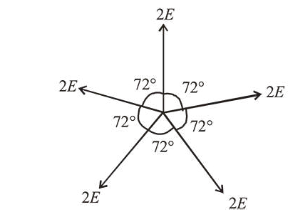# Ten charges are placed on the circumference of a circle of radius R with constant angular separation between successive charges.

Question:

Ten charges are placed on the circumference of a circle of radius $R$ with constant angular separation between successive charges. Alternate charges $1,3,5,7,9$ have charge $(+q)$ each, while $2,4,6,8,10$ have charge $(-q)$ each. The potential $V$ and the electric field $E$ at the centre of the circle are respectively:

(Take $V=0$ at infinity)

1. (1) $V=\frac{10 q}{4 \pi \varepsilon_{0} R} ; E=0$

2. (2) $V=0 ; E=\frac{10 q}{4 \pi \varepsilon_{0} R^{2}}$

3. (3) $V=0 ; E=0$

4. (4) $V=\frac{10 q}{4 \pi \varepsilon_{0} R} ; E=\frac{10 q}{4 \pi \varepsilon_{0} R^{2}}$

Correct Option: , 3

Solution:

(3) Potential at the centre, $V_{C}=\frac{K Q_{\text {net }}}{\mathrm{R}}$

$\because Q_{\text {net }}=0$

$\therefore V_{C}=0$

Let $E$ be electric field produced by each charge at the centre, then resultant electric field will be $E_{C}=0$, since equal electric field vectors are acting at equal angle so their resultant is equal to zero.